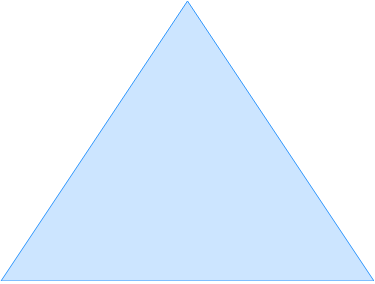# Tessellations using rotations

### Tessellations using rotations

We have a square tile on the floor, and we rotate it around its center. When it is turned 90°, you can see the tile in its original place as if it has never moved at all. Then, we can conclude that the square tile has a rotational symmetry of 90°. In the previous section, we have learned that some shapes can reflect by flipping them over. The number of ways that a particular shape can reflect is dictated by the number of axes of reflection symmetry it has. We will learn how to find out that number of axes of symmetry in this section.

#### Lessons

• 1.
Name a polygon that has a rotational symmetry of:
a)
30°

b)
45°

c)
120°

d)
180°

• 2.
Find the axe(s) of reflection symmetry for the polygons below.
a)b)c)d)